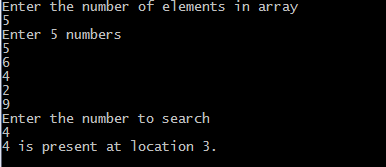## Print this Job Post# Linear search in c

## Linear search c program

#include <stdio.h>
#include<conio.h>
void main()
{
int array, search, c, n;
clrscr();
printf("Enter the number of elements in array\n");
scanf("%d",&n);
printf("Enter %d integer(s)\n", n);
for (c = 0; c < n; c++)
scanf("%d", &array[c]);
printf("Enter the number to search\n");
scanf("%d", &search);
for (c = 0; c < n; c++)
{
if (array[c] == search)     /* if required element found */
{
printf("%d is present at location %d.\n", search, c+1);
break;
}
}
if (c == n)
printf("%d is not present in array.\n", search);
getch();
}

## Linear search for multiple occurrences

In the code below we will print all the locations at which required element is found and also the number of times it occur in the list.
#include <stdio.h>
#include<conio.h>
void main()
{
int array, search, c, n, count = 0;
clrscr();
printf("Enter the number of elements in array\n");
scanf("%d",&n);
printf("Enter %d numbers\n", n);
for ( c = 0 ; c < n ; c++ )
scanf("%d",&array[c]);
printf("Enter the number to search\n");
scanf("%d",&search);
for ( c = 0 ; c < n ; c++ )
{
if ( array[c] == search )
{
printf("%d is present at location %d.\n", search, c+1);
count++;
}
}
if ( count == 0 )
printf("%d is not present in array.\n", search);
else
printf("%d is present %d times in array.\n", search, count);
getch();
}## C program for linear search using function

#include <stdio.h>
#include<conio.h>
int linear_search(int*, int, int);
void main()
{
int array, search, c, n, position;
clrscr();
printf("Enter the number of elements in array\n");
scanf("%d",&n);
printf("Enter %d numbers\n", n);
for ( c = 0 ; c < n ; c++ )
scanf("%d", &array[c]);
printf("Enter the number to search\n");
scanf("%d",&search);
position = linear_search(array, n, search);
if ( position == -1 )
printf("%d is not present in array.\n", search);
else
printf("%d is present at location %d.\n", search, position+1);
getch();
int linear_search(int *pointer, int n, int find)
{
int c;
for ( c = 0 ; c < n ; c++ )
{
if ( *(pointer+c) == find )
return c;
}
return -1;
}